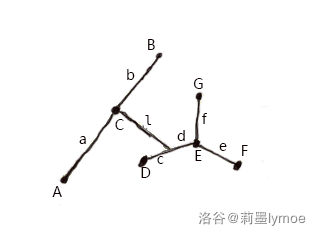Part 0-题目分析

part 1-测试点 1~10 40 分

part 2-测试点 15~20 64 分（40+24）

part 3-测试点 11~14 16 分

$\because dis(a_1,a_2)\ge k$

$\therefore dis(a_2,a_x) < k$

$\because dis(a_2,a_3) \ge k ,dis(a_2,a_x)<k$

$dis(a_3,a_x)\ge k,dis(a_1,a_3)<k$

$\because dis(a_1,a_2)\ge k,a_1\ge1$

$\therefore dis(1,a_2)\ge k$

$\because dis(a_2,a_3)\ge k,a_3\le n$

$\therefore dis(a_2,n) \ge k$

part 4-测试点 21~25 100 分

$\because dis(a_1,a_2)\ge k$

$\therefore dis(a_2,a_x) < k$

$\because dis(a_2,a_3) \ge k ,dis(a_2,a_x)<k$

$\therefore dis(a_3,a_x)\ge k,dis(a_1,a_3)<k$

…（若比较难理解可以自行画图）$AB$ 为树的直径， $(D,F,G)$ 为直径外一三元环。

$(f+d)+c \ge k$

$\therefore b+l<c$

$\therefore a+l+c>a+l+b+l\ge a+b$

#include<stdio.h>
#include<string.h>
#define MAXN 1000005
struct node
{
int eh;
}V[MAXN];
struct edge
{
int v;
int to;
int next;
}E[MAXN*2];
int tot;
int dis1[MAXN],dis2[MAXN];
{
E[++tot]=(edge){v,to,V[from].eh};
V[from].eh=tot;
}
void dfs(int i,int fa,int* dis)
{
int p;
for(p=V[i].eh;p;p=E[p].next)
{
if(E[p].to==fa) continue;
dis[E[p].to]=dis[i]+E[p].v;
dfs(E[p].to,i,dis);
}
}
int main()
{
int n,i,T,x,y,v,k,flag;
scanf("%d",&T);
while(T--)
{
memset(V,0,sizeof(V));
memset(E,0,sizeof(E));
tot=0;flag=1;
scanf("%d%d",&n,&k);
for(i=0;i<n-1;i++)
{
scanf("%d%d%d",&x,&y,&v);
}
dis1=0;
dfs(1,0,dis1);x=1;
for(i=2;i<=n;i++)
{
if(dis1[i]>dis1[x]) x=i;
}
dis1[x]=0;
dfs(x,0,dis1);y=x;
for(i=1;i<=n;i++)
{
if(dis1[i]>dis1[y]) y=i;
}
dis2[y]=0;
dfs(y,0,dis2);
for(i=1;i<=n;i++)
{
if(dis1[i]>=k&&dis2[i]>=k) flag=0;
}
if(flag) printf("Yes\n");
else printf("Baka Chino\n");
}
return 0;
}


### 1 条评论orzorz 书虫太强了